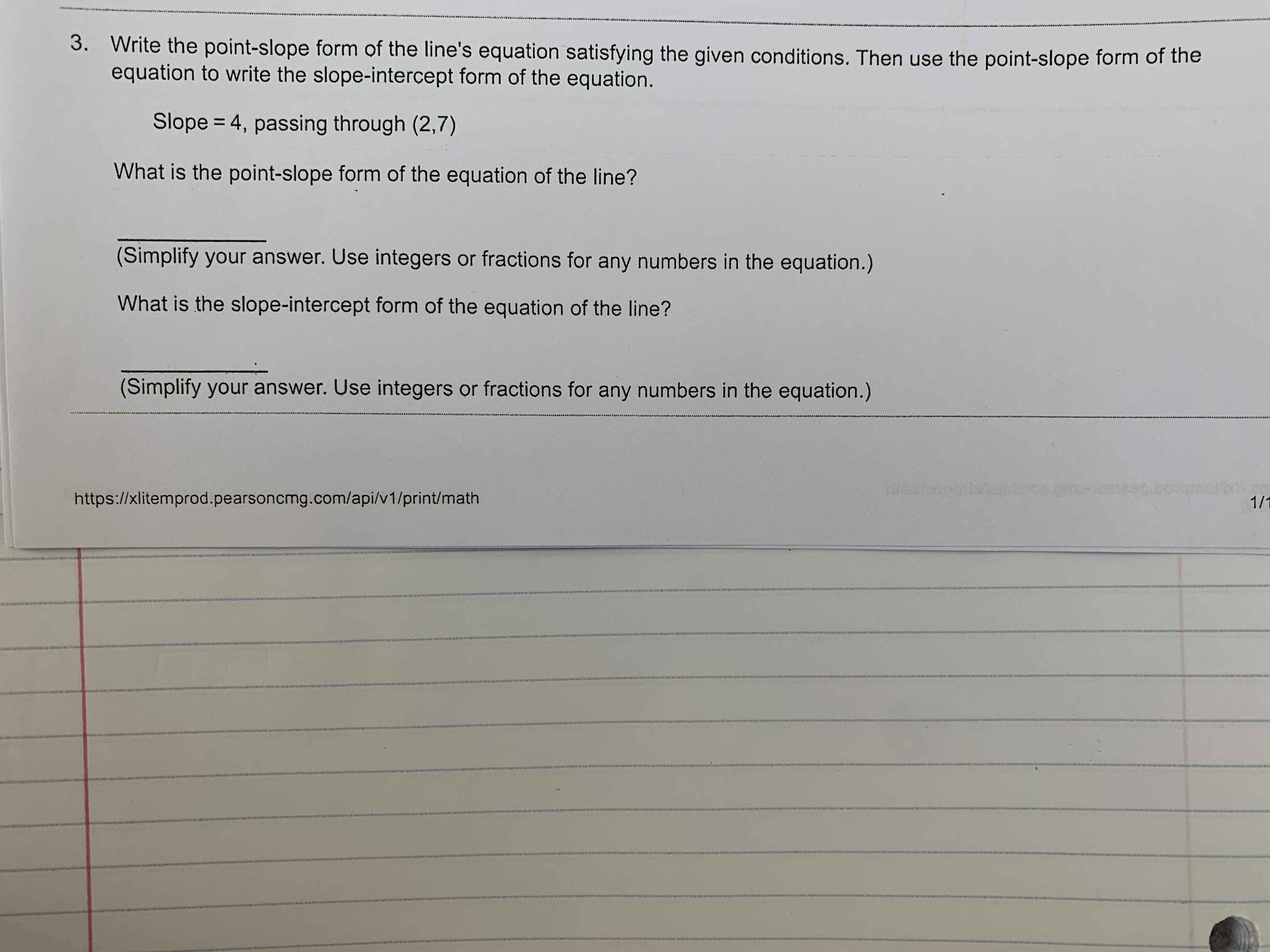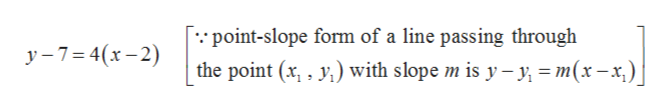# wwn wwwww www3.Write the point-slope form of the line's equation satisfying the given conditions. Then use the point-slope form of theequation to write the slope-intercept form of the equation.Slope = 4, passing through (2,7)What is the point-slope form of the equation of the line?(Simplify your answer. Use integersor fractions for any numbers in the equation.)What is the slope-intercept form of the equation of the line?(Simplify your answer. Use integers or fractions for any numbers in the equation.)1/1https://xlitemprod.pearsoncmg.com/api/v1/print/math

Question
4 views

Can someone help me with this problem?help_outlineImage Transcriptionclosewwn www ww www 3. Write the point-slope form of the line's equation satisfying the given conditions. Then use the point-slope form of the equation to write the slope-intercept form of the equation. Slope = 4, passing through (2,7) What is the point-slope form of the equation of the line? (Simplify your answer. Use integers or fractions for any numbers in the equation.) What is the slope-intercept form of the equation of the line? (Simplify your answer. Use integers or fractions for any numbers in the equation.) 1/1 https://xlitemprod.pearsoncmg.com/api/v1/print/math fullscreen
check_circle

Step 1

Given,

Step 2

Point-slope form of the equation o...help_outlineImage Transcriptionclose. point-slope form of a line passing through y-7 4(x-2) the point (x y with slope m is y-ym(x-x.) fullscreen

### Want to see the full answer?

See Solution

#### Want to see this answer and more?

Solutions are written by subject experts who are available 24/7. Questions are typically answered within 1 hour.*

See Solution
*Response times may vary by subject and question.
Tagged in

### Other